Courses

# BASIC CONCEPTS OF DIFFERENTIAL AND INTEGRAL CALCULUS MCQ Test - 3

## 40 Questions MCQ Test | BASIC CONCEPTS OF DIFFERENTIAL AND INTEGRAL CALCULUS MCQ Test - 3

Description
This mock test of BASIC CONCEPTS OF DIFFERENTIAL AND INTEGRAL CALCULUS MCQ Test - 3 for Quant helps you for every Quant entrance exam. This contains 40 Multiple Choice Questions for Quant BASIC CONCEPTS OF DIFFERENTIAL AND INTEGRAL CALCULUS MCQ Test - 3 (mcq) to study with solutions a complete question bank. The solved questions answers in this BASIC CONCEPTS OF DIFFERENTIAL AND INTEGRAL CALCULUS MCQ Test - 3 quiz give you a good mix of easy questions and tough questions. Quant students definitely take this BASIC CONCEPTS OF DIFFERENTIAL AND INTEGRAL CALCULUS MCQ Test - 3 exercise for a better result in the exam. You can find other BASIC CONCEPTS OF DIFFERENTIAL AND INTEGRAL CALCULUS MCQ Test - 3 extra questions, long questions & short questions for Quant on EduRev as well by searching above.
QUESTION: 1

Solution:
QUESTION: 2

Solution:
QUESTION: 3

### If y = logyx , then  dy/dx is equal to:

Solution:
QUESTION: 4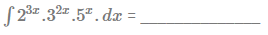Solution:
QUESTION: 5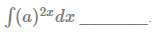Solution:
QUESTION: 6

If y = ea logx + ex loga, then dy/dx=

Solution:
QUESTION: 7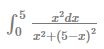is equal to __________.

Solution:
QUESTION: 8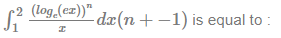Solution:
QUESTION: 9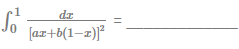Solution:
QUESTION: 10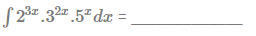Solution:
QUESTION: 11

If x = log t , y = et, then dy/dx =

Solution:
QUESTION: 12

If x3 - 2x2 y2 + 5x + y - 5 = 0 then dy/dx at x = 1 , y = 1 is equal to

Solution:
QUESTION: 13

If y = log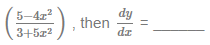Solution:
QUESTION: 14

If y = aenx + be-nx, then d2y/dx2 is equal to _________.

Solution:
QUESTION: 15

The points on the curve y = x3 - x2 - x + 1where the tangent is parallel to x -axis are

Solution:
QUESTION: 16

The equation of the tangent to the curve, f = x2 - 3x + 2, at the point (2, 7) is-

Solution:
QUESTION: 17

Solve :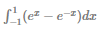Solution:
QUESTION: 18

Integrate w.r.t x, (x-x3)-1

Solution:
QUESTION: 19

If f'(x) = 3x2 2/x3 , f(1) = 0 and f(x) = ___________.

Solution:
QUESTION: 20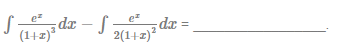Solution:
QUESTION: 21

Integrate w.r.t x, (2x+3)1/2 form lower limit 3 to upper limit 11 of x

Solution:
QUESTION: 22

If x = x t, y = c/t, then dy/dx is equal to:

Solution:
QUESTION: 23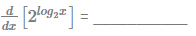Solution:
QUESTION: 24

If f(x) = xC3; then f'(1) = ?

Solution:
QUESTION: 25

Integrate w.r.t x, (25-x2)-1 from lower limit 3 to upper limit 4 of x

Solution:
QUESTION: 26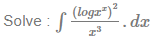Solution:
QUESTION: 27

Integrate w.r.t x, x3[(x-a)(x-b)(x-c)]-1 given that
1/A = (a-b)(a-c)/a3,1/B=(b-a)(b-c)/b3, 1/C=(c-a)(c-b)/c3

Solution:
QUESTION: 28

If g(x) =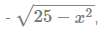then  limx→1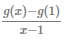is equal to _______.

Solution:
QUESTION: 29

Integrate w.r.t x, x2e3x

Solution:
QUESTION: 30

Integrate w.r.t x, xex(x+1)-2

Solution:
QUESTION: 31

Itegrate w.r.t x, xex

Solution:
QUESTION: 32

Integrate w.r.t x, logx

Solution:
QUESTION: 33

Integrate w.r.t x, (x+1)(3+2x-x2)-1 + c

Solution:
QUESTION: 34

Integrate w.r.t x, (5x2+8x+4)-1/2

Solution:
QUESTION: 35

Integrate w.r.t x, xnlogx

Solution:
QUESTION: 36

Integrate w.r.t x, x2ex

Solution:
QUESTION: 37

Integrate w.r.t x, x(x-1)-1(2x+1)-1

Solution:
QUESTION: 38

Integrate w.r.t x, x(x2+4)5

Solution:
QUESTION: 39

Integrate w.r.t x, x-3[4x6 + 3x5+2x4+x3+x2+ 1]

Solution:
QUESTION: 40

Integrate w.r.t x, x(x2+3)-2

Solution: